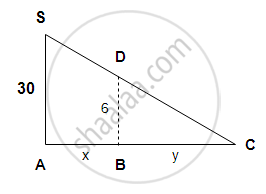# A Point Source of Light is Hung 30 Feet Directly Above a Straight Horizontal Path on Which a Man of 6 Feet in Height is Walking. - Mathematics and Statistics

Sum

A point source of light is hung 30 feet directly above a straight horizontal path on which a man of 6 feet in height is walking. How fast will the man’s shadow lengthen and how fast will the tip of shadow move when he is walking away from the light at the rate of 100 ft/min.

#### SolutionLet S be the position of source of light.

Let BD be the position of the man at a time t.

Let AB = x and BC = length of the shadow = y

Now

dx/dt=100

From the ∆ASC ~ ∆BDC

:.(AS)/(BD)=(AC)/(BC)

30/6=(x+y)/y

∴ 5y =  x+ y

4y = x

4 "dy"/"dt" = "dx"/"dt"

:.dy/dt =1/4(100)=25

∴ The shadow of the man is lengthening at the rate 25 ft/min

The tip of shadow is at C. Let AC = z.

∴ AB = c

(AS)/(BD)=(AC)/(BC)

∴ BC = z – x

:.30/6=z/(z-x)

5z – 5x = z

4z = 5x

4  "dz"/"dt" = 5  "dx"/"dt"

4  "dz"/"dt" = 5 xx 100

"dz"/"dt" = 5 xx 25 = 125

The tip of the shadow is moving at the rate 125 ft/min.

Concept: Rate of Change of Bodies Or Quantities
Is there an error in this question or solution?